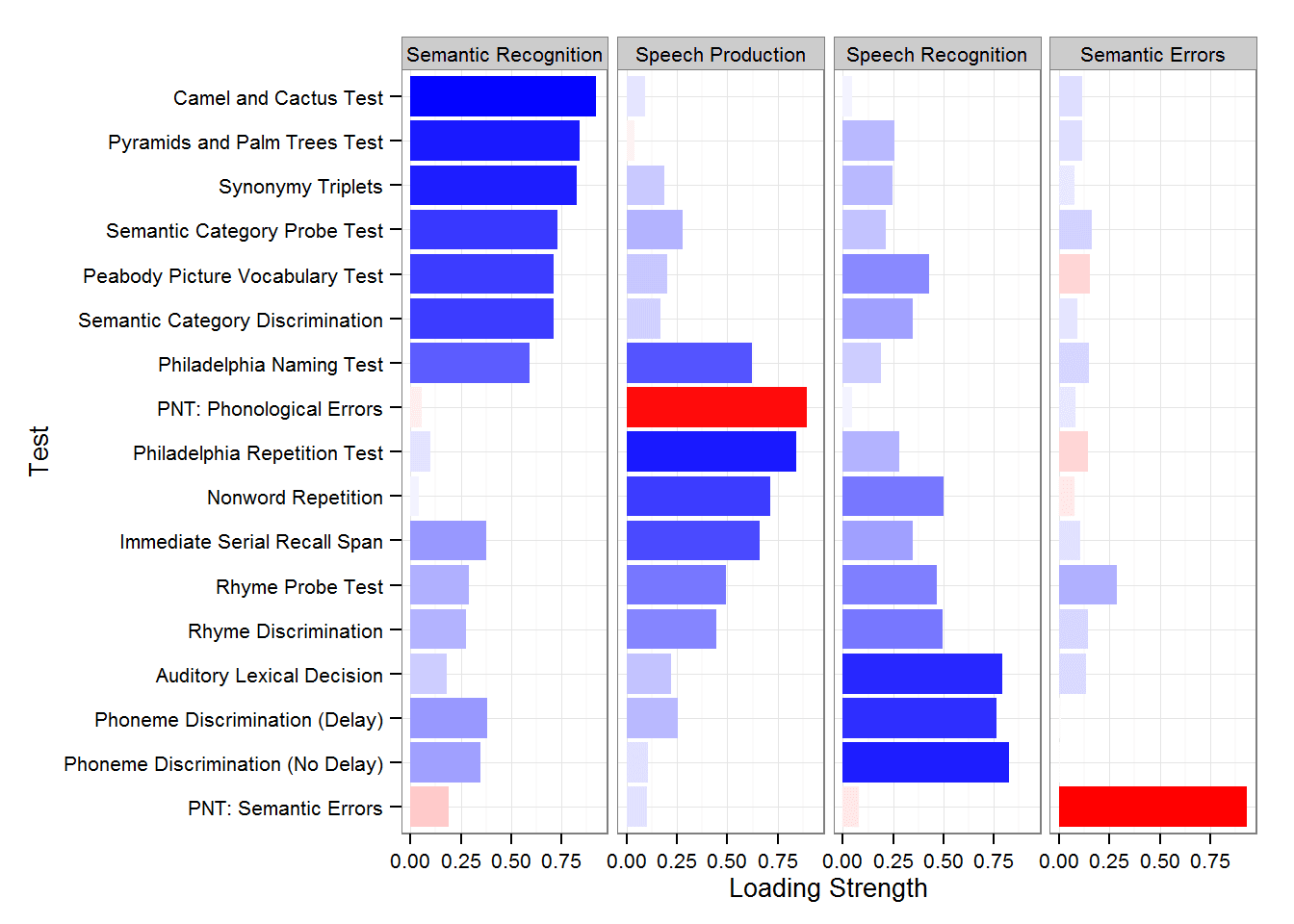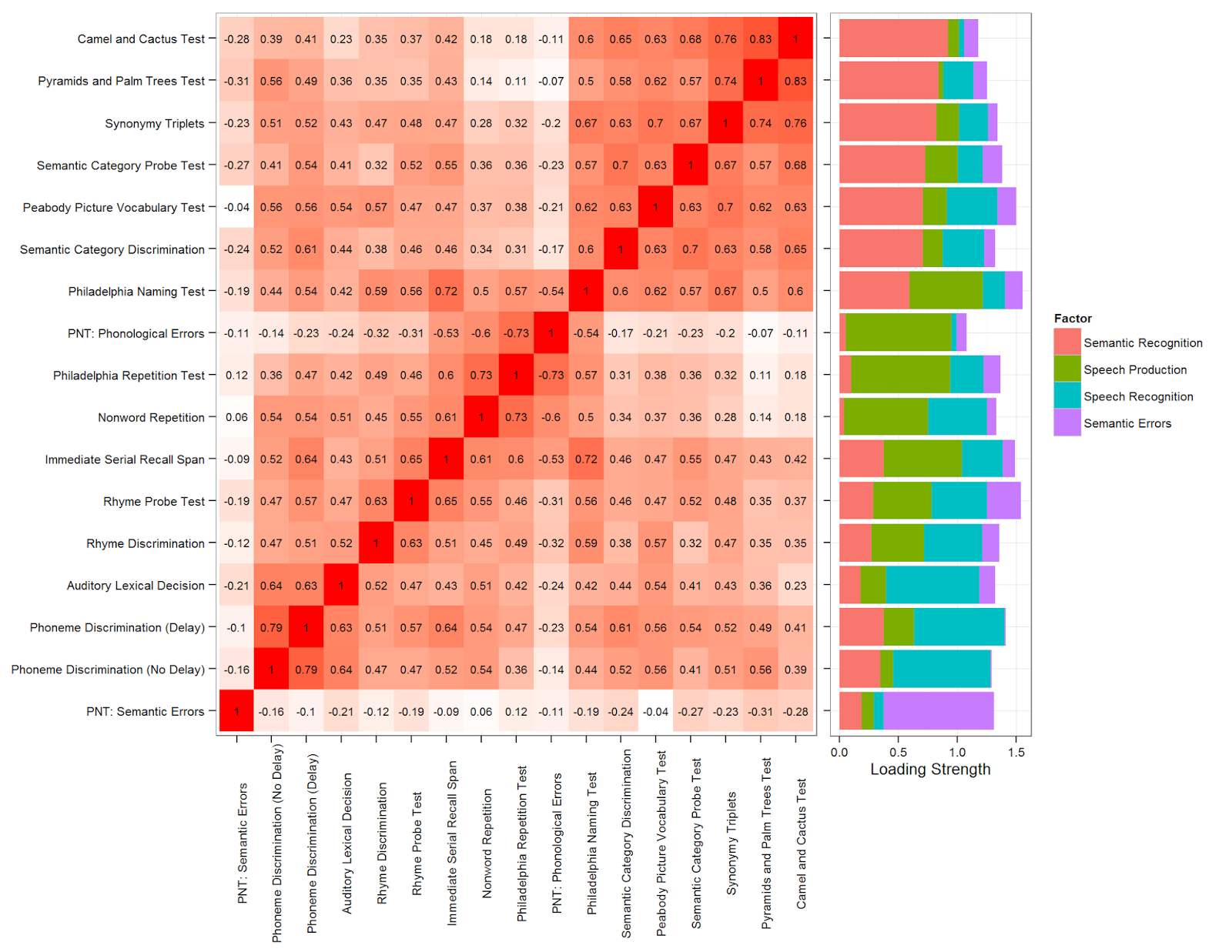## Monday, April 20, 2015

### Plotting Factor Analysis Results

A recent factor analysis project (as discussed previously here, here, and here) gave me an opportunity to experiment with some different ways of visualizing highly multidimensional data sets. Factor analysis results are often presented in tables of factor loadings, which are good when you want the numerical details, but bad when you want to convey larger-scale patterns – loadings of 0.91 and 0.19 look similar in a table but very different in a graph. The detailed code is posted on RPubs because embedding the code, output, and figures in a webpage is much, much easier using RStudio's markdown functions. That version shows how to get these example data and how to format them correctly for these plots. Here I will just post the key plot commands and figures those commands produce.

First, a bar graph showing each measure's factor loadings with each factor in a separate facet (subplot):

```#For each test, plot the loading as length and fill color of a bar
# note that the length will be the absolute value of the loading but the
# fill color will be the signed value, more on this below
facet_wrap(~ Factor, nrow=1) + #place the factors in separate facets
geom_bar(stat="identity") + #make the bars
coord_flip() + #flip the axes so the test names can be horizontal
#define the fill color gradient: blue=positive, red=negative
high = "blue", mid = "white", low = "red",
midpoint=0, guide=F) +
theme_bw(base_size=10) #use a black-and-white theme with set font size```
Created by Pretty R at inside-R.orgFig. 1 from Mirman et al., 2015, Nature Communications
Second, the full pairwise correlation matrix with a stacked bar graph showing each measure's (absolute) loading on each factor:

```library(grid) #for adjusting plot margins
#place the tests on the x- and y-axes,
#fill the elements with the strength of the correlation
p1 <- ggplot(corrs.m, aes(Test2, Test, fill=abs(Correlation))) +
geom_tile() + #rectangles for each correlation
#add actual correlation value in the rectangle
geom_text(aes(label = round(Correlation, 2)), size=2.5) +
theme_bw(base_size=10) + #black and white theme with set font size
#rotate x-axis labels so they don't overlap,
#get rid of unnecessary axis titles
theme(axis.text.x = element_text(angle = 90),
axis.title.x=element_blank(),
axis.title.y=element_blank(),
plot.margin = unit(c(3, 1, 0, 0), "mm")) +

geom_bar(stat="identity") + coord_flip() +
#remove labels and tweak margins for combining with the correlation matrix plot
theme(axis.text.y = element_blank(),
axis.title.y = element_blank(),
plot.margin = unit(c(3,1,39,-3), "mm"))
library(gridExtra) #for combining the two plots
grid.arrange(p1, p2, ncol=2, widths=c(2, 1)) #side-by-side, matrix gets more space```
Created by Pretty R at inside-R.orgFig. 2 from Mirman et al., in press, Neuropsychologia

#### 1 comment:

1.Neat visualizations! You might also be interested in tableplots - they are very useful for interpreting factor analysis patterns, either with or without shading (R manual: http://cran.r-project.org/web/packages/tableplot/tableplot.pdf and brief article: http://www.datavis.ca/papers/Tableplot-Kwan-etal2009.pdf)